# Lions Weekly: February 16, 2022# A Note From Mr. Sandefur

Lion Family,

I want to thank all of those who were involved in this year’s Love Gala. Last Saturday night was an incredible night for the Academy as we were able to celebrate our teachers, former administrators, and those who have made a lasting impact on TKA. The evening was full of fellowship and generosity as we sought to honor the Lord and all who have made a difference for TKA. We look forward to announcing projects soon that will be funded by the evening as we celebrate the generosity of all involved.

I personally would like to invite you to circle the date of Saturday, March 5th, on your calendars as we host “Forged” Parent Conference. This conference has been uniquely designed for our families as we link arms together in biblical training regarding parenting in a post-Christian society. At the heart of all we do at The King’s Academy is a deeply rooted biblical worldview, and this event is no different. Our prayer is that our families and our community are blessed by the time of worship, biblical teaching, and fellowship that will be present. Please prayerfully consider making Forged a must attend event for you and your family.

In Christ Alone,

Jeremy Sandefur
President

 table div table+table+table+table div table{width:100%;padding:0}table div table+table+table+table div table img{width:96.23%;padding:0;float:none}table div table+table+table+table div table td{width:100%;padding:0 1.88% 18px}/* styles */# Monday, February 21

TKA school and offices will be closed on Monday, February 21, in observation of Presidents Day.

 table div table+table+table+table+table+table div table{width:100%;padding:0}table div table+table+table+table+table+table div table img{width:96.23%;padding:0;float:none}table div table+table+table+table+table+table div table td{width:100%;padding:0 1.88% 18px}/* styles */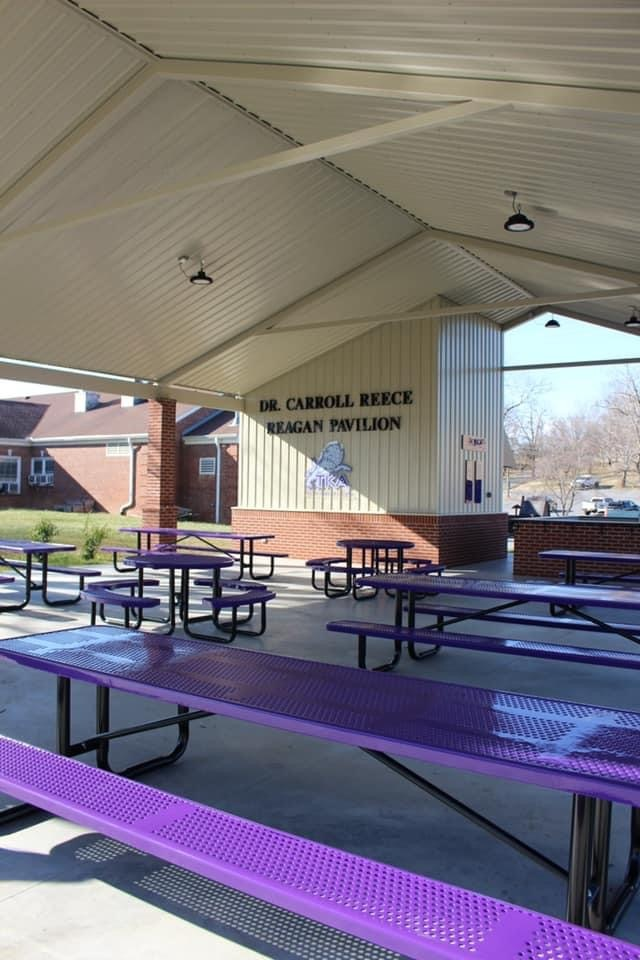# Dr. Carroll Reece Reagan Pavilion

It has been an exciting week on campus as the picnic tables and benches arrived for the Dr. Carroll Reece Reagan Pavilion! Thank you to all the families, individuals, and companies who graciously gave to help make this happen. We are looking forward to using this space for many years to come!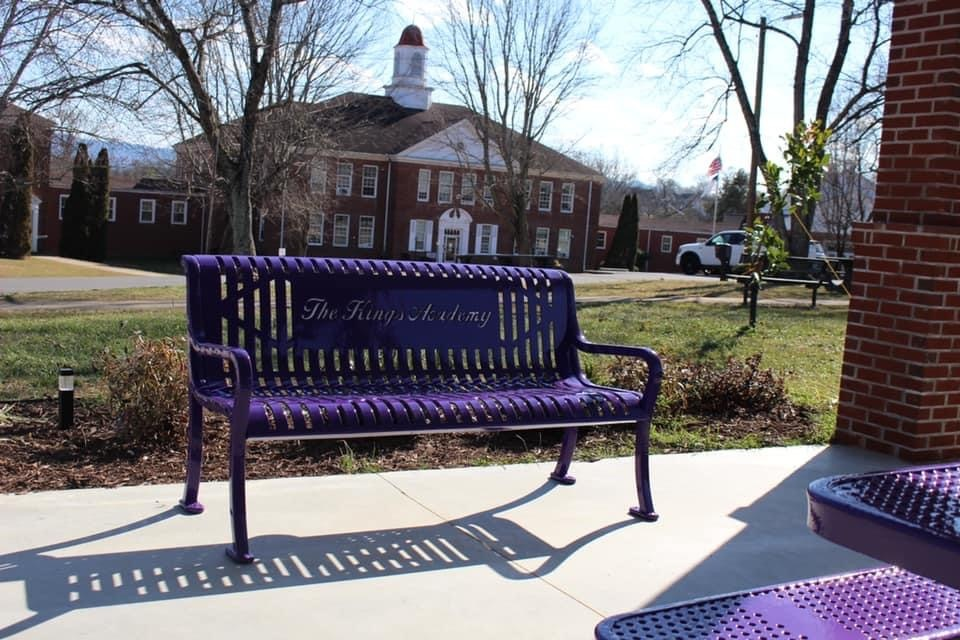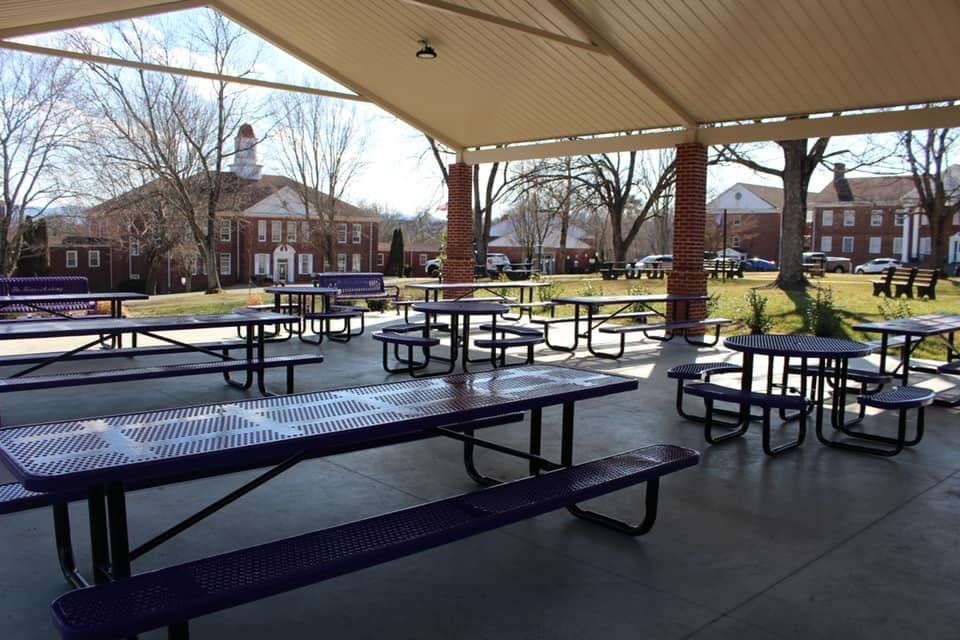table div table+table+table+table+table+table+table+table+table div table{width:100%;padding:0}table div table+table+table+table+table+table+table+table+table div table img{width:96.23%;padding:0;float:none}table div table+table+table+table+table+table+table+table+table div table td{width:100%;padding:0 1.88% 18px}/* styles */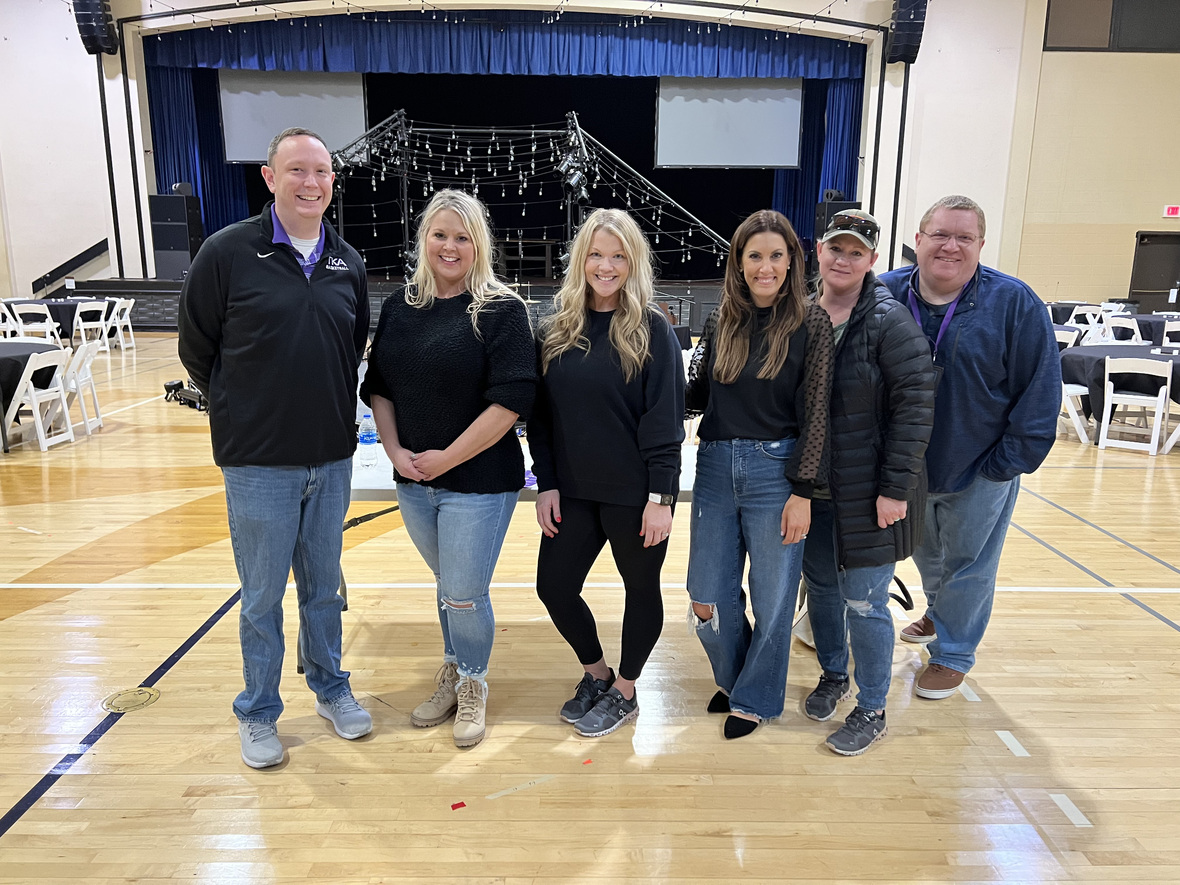# Love Gala

Thank you to all who attended the Love Gala and participated in the auctions. We are excited about the ways this will help the PTF Love on the Academy faculty, students and facilities.

A special thank you to our PTF who hosted this wonderful event!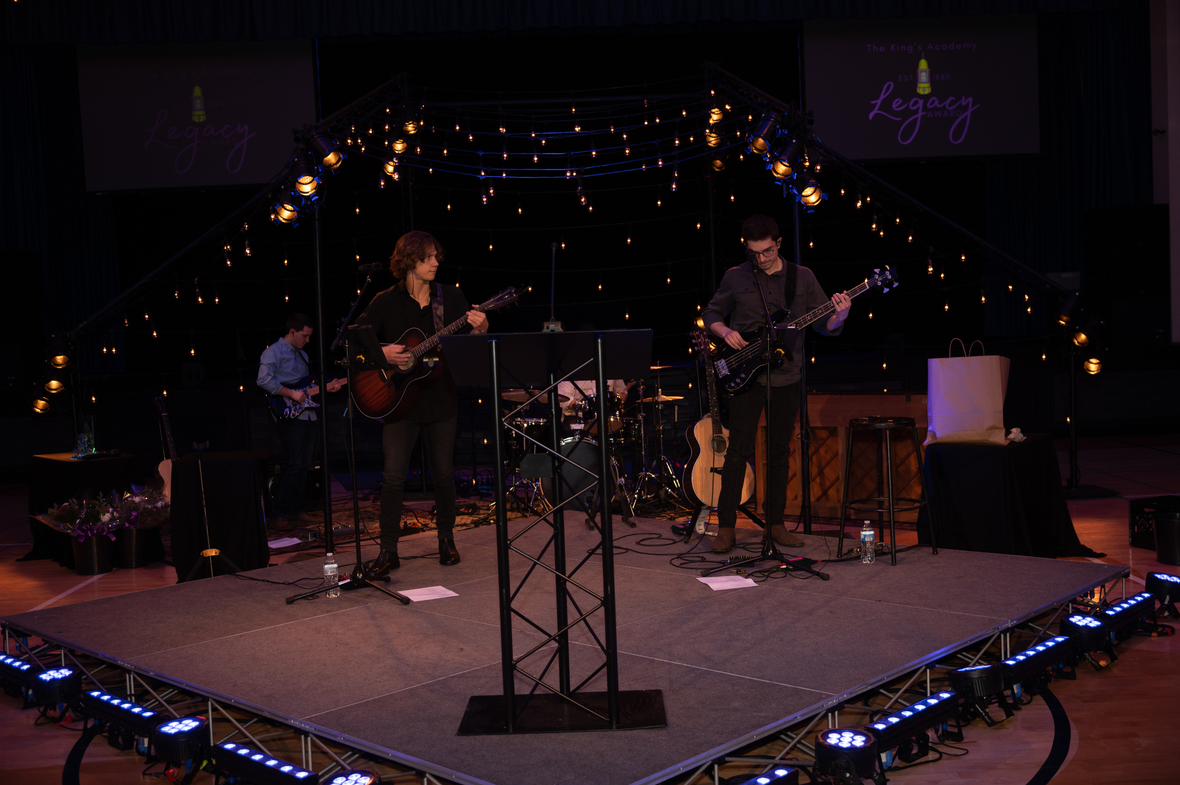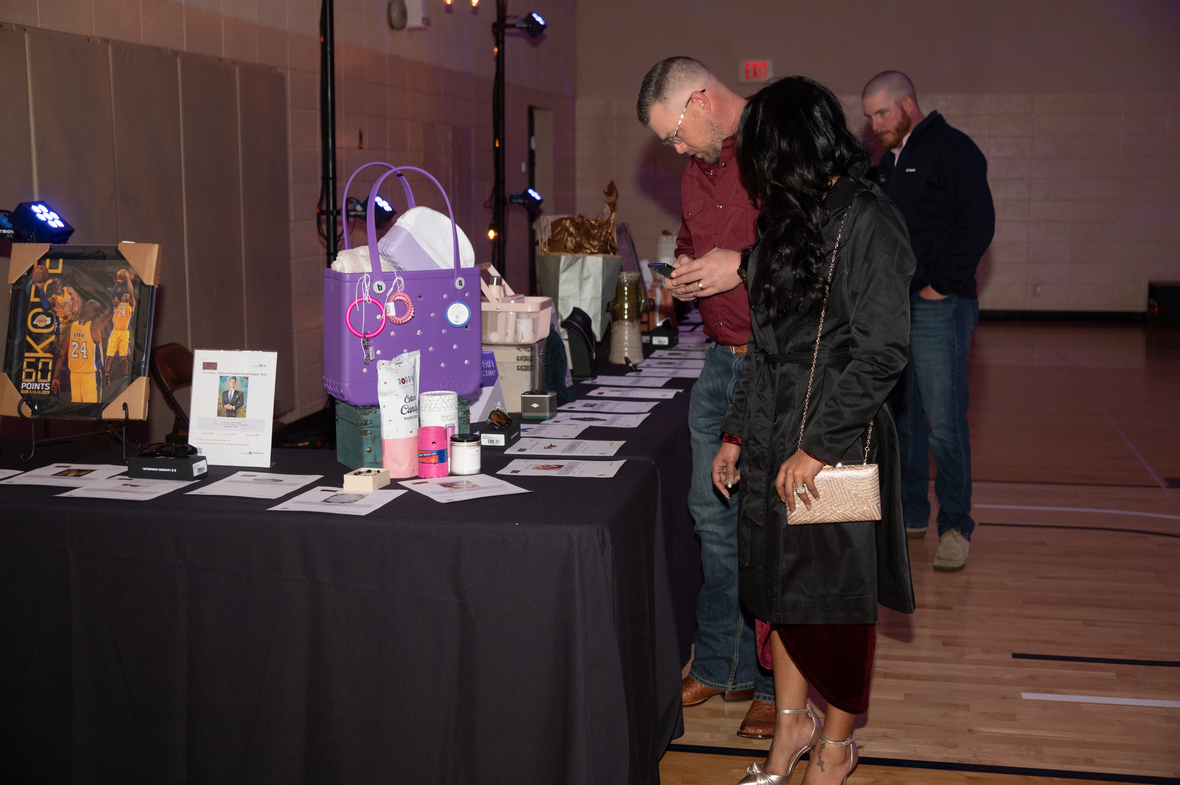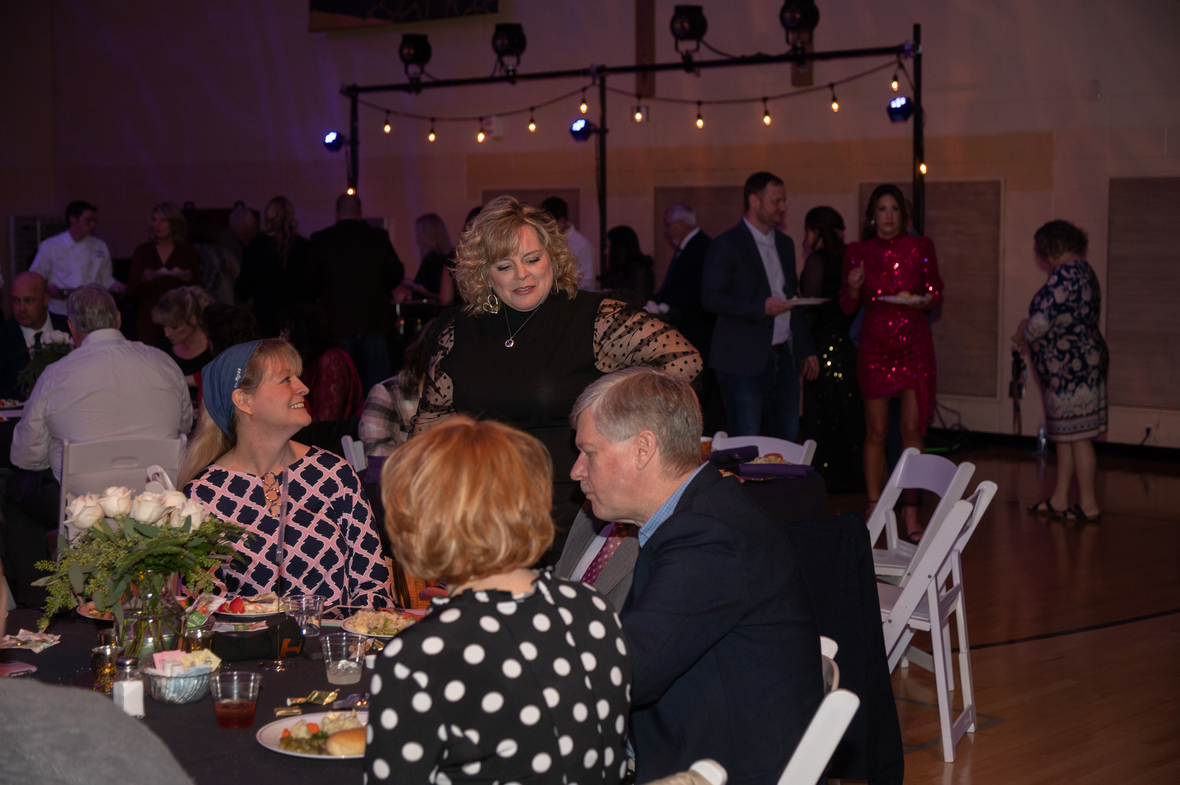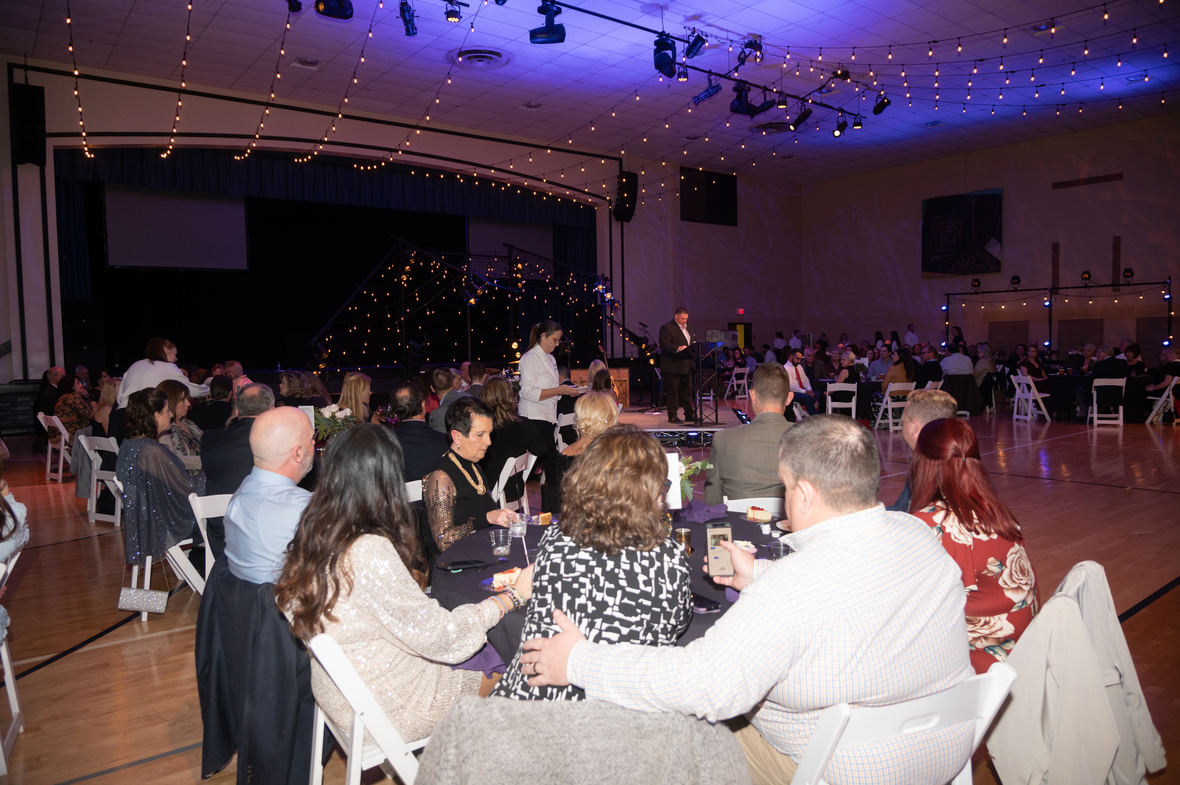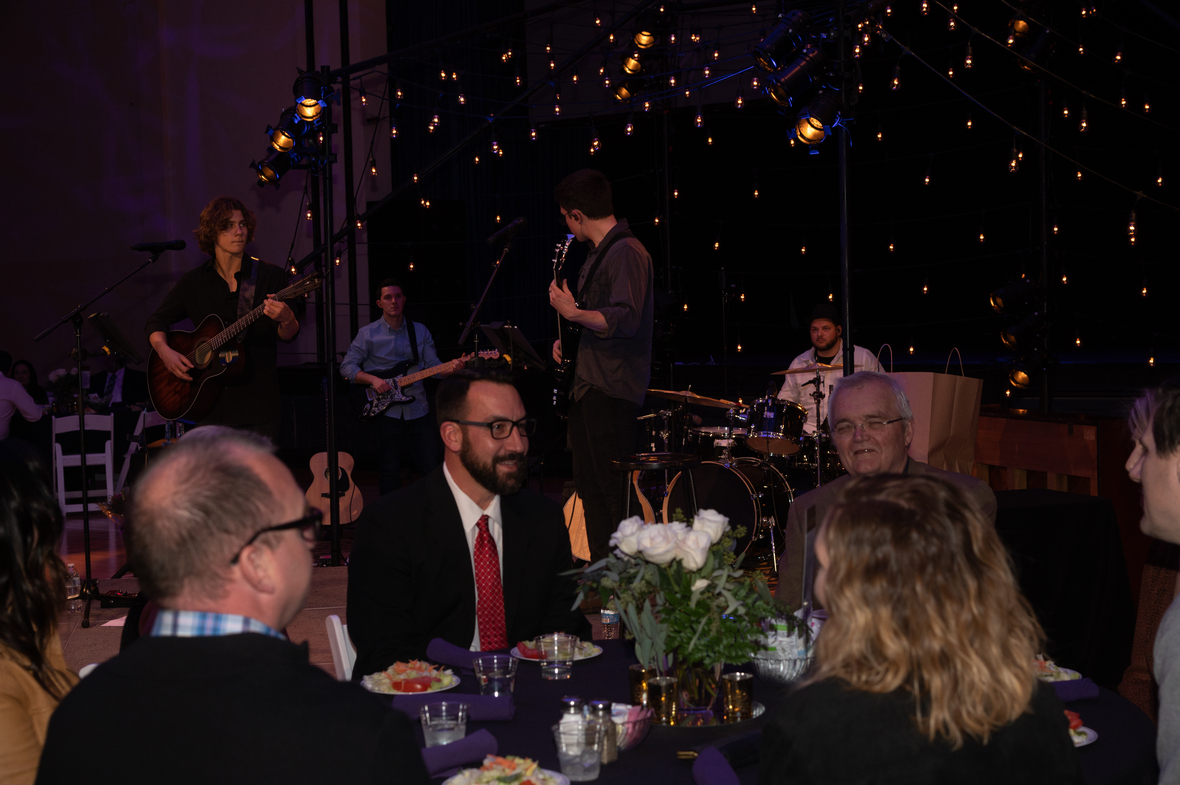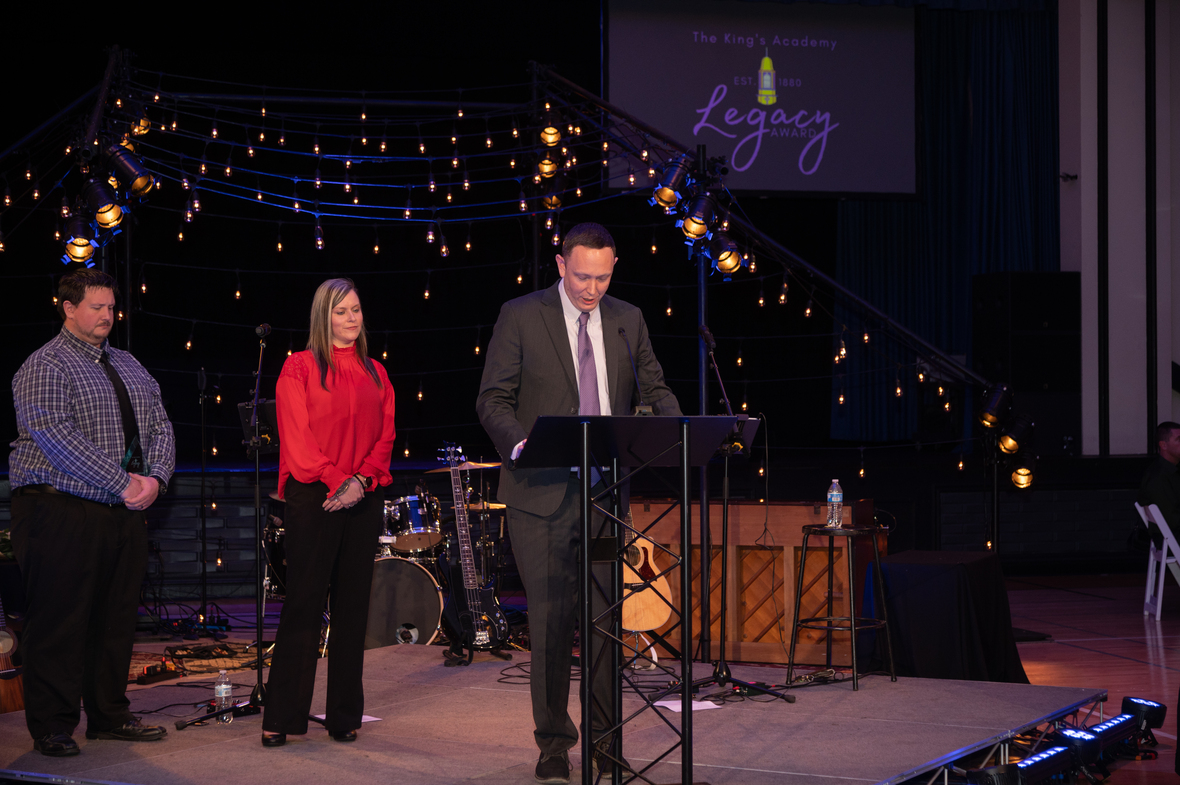table div table+table+table+table+table+table+table+table+table+table+table+table+table+table div table{width:100%;padding:0}table div table+table+table+table+table+table+table+table+table+table+table+table+table+table div table img{width:96.23%;padding:0;float:none}table div table+table+table+table+table+table+table+table+table+table+table+table+table+table div table td{width:100%;padding:0 1.88% 18px}/* styles */# 2021-2022 Teacher of the year

We at TKA are blessed to have an amazing teaching faculty. Each one of our teachers goes above and beyond on a daily basis to show the love of Christ to our Lions as they seek to develop servant leaders for Christ.

This year's Elementary Teacher of the Year is Mrs. Carole Daft. The Middle/High School Teacher of the year is Mr. Lee Daft. We are thankful for both of these teachers and all they bring to TKA.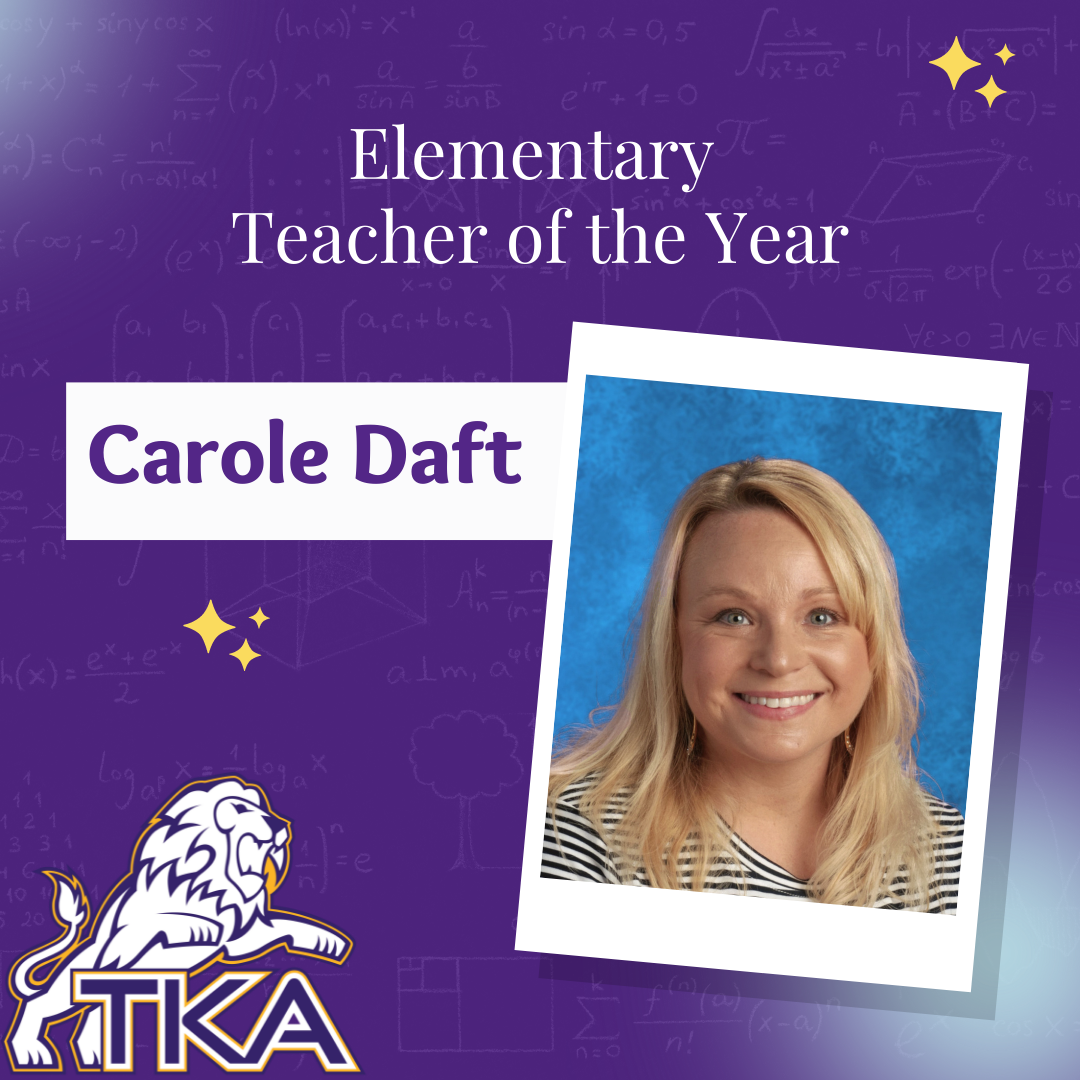table div table+table+table+table+table+table+table+table+table+table+table+table+table+table+table+table+table div table{width:100%;padding:0}table div table+table+table+table+table+table+table+table+table+table+table+table+table+table+table+table+table div table img{width:96.23%;padding:0;float:none}table div table+table+table+table+table+table+table+table+table+table+table+table+table+table+table+table+table div table td{width:100%;padding:0 1.88% 18px}/* styles */# 2021-2022 Legacy Award Recipients

In the 142 years that TKA has been an academic institution, many people have made a lasting impact and left a legacy for generations to come. The Legacy Award was established this year to honor those who have left a Legacy at TKA. This year we had two recipients of the award.

The first award was given to Mrs. Brenda Ogle Burchfield who has continued her family's love and generous giving to TKA in order to ensure that the future of the Academy is as rich as its' heritage.

The second recipient was given to Dr. Walt Grubb who served as an administrator at TKA for over thirty years. Dr. Grubb faithfully served throughout his career, leading the Academy into the college preparatory model of which TKA excels in today.

We are thankful for both of these incredible people and the legacy they have left for The King's Academy.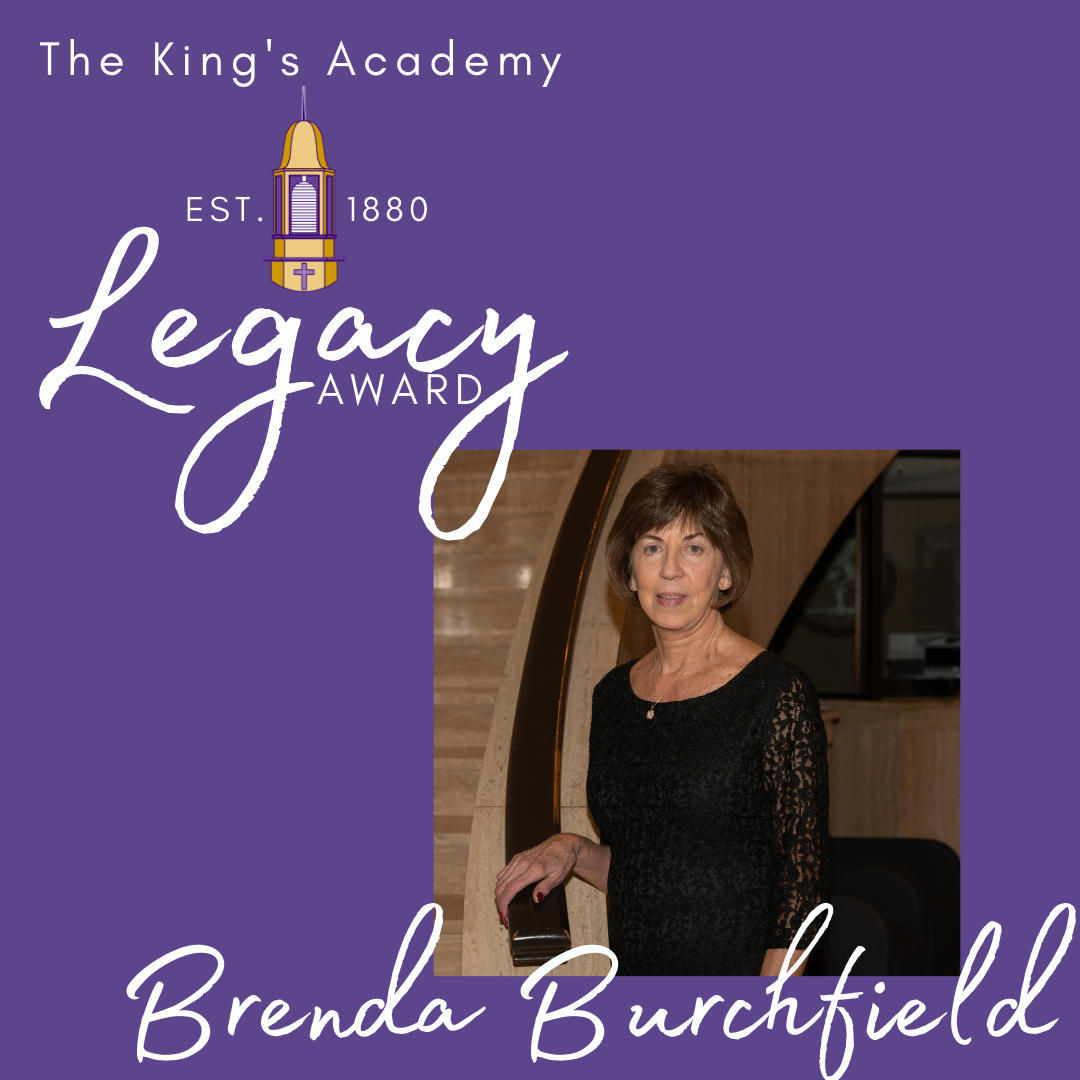table div table+table+table+table+table+table+table+table+table+table+table+table+table+table+table+table+table+table+table+table div table{width:100%;padding:0}table div table+table+table+table+table+table+table+table+table+table+table+table+table+table+table+table+table+table+table+table div table img{width:96.23%;padding:0;float:none}table div table+table+table+table+table+table+table+table+table+table+table+table+table+table+table+table+table+table+table+table div table td{width:100%;padding:0 1.88% 18px}/* styles */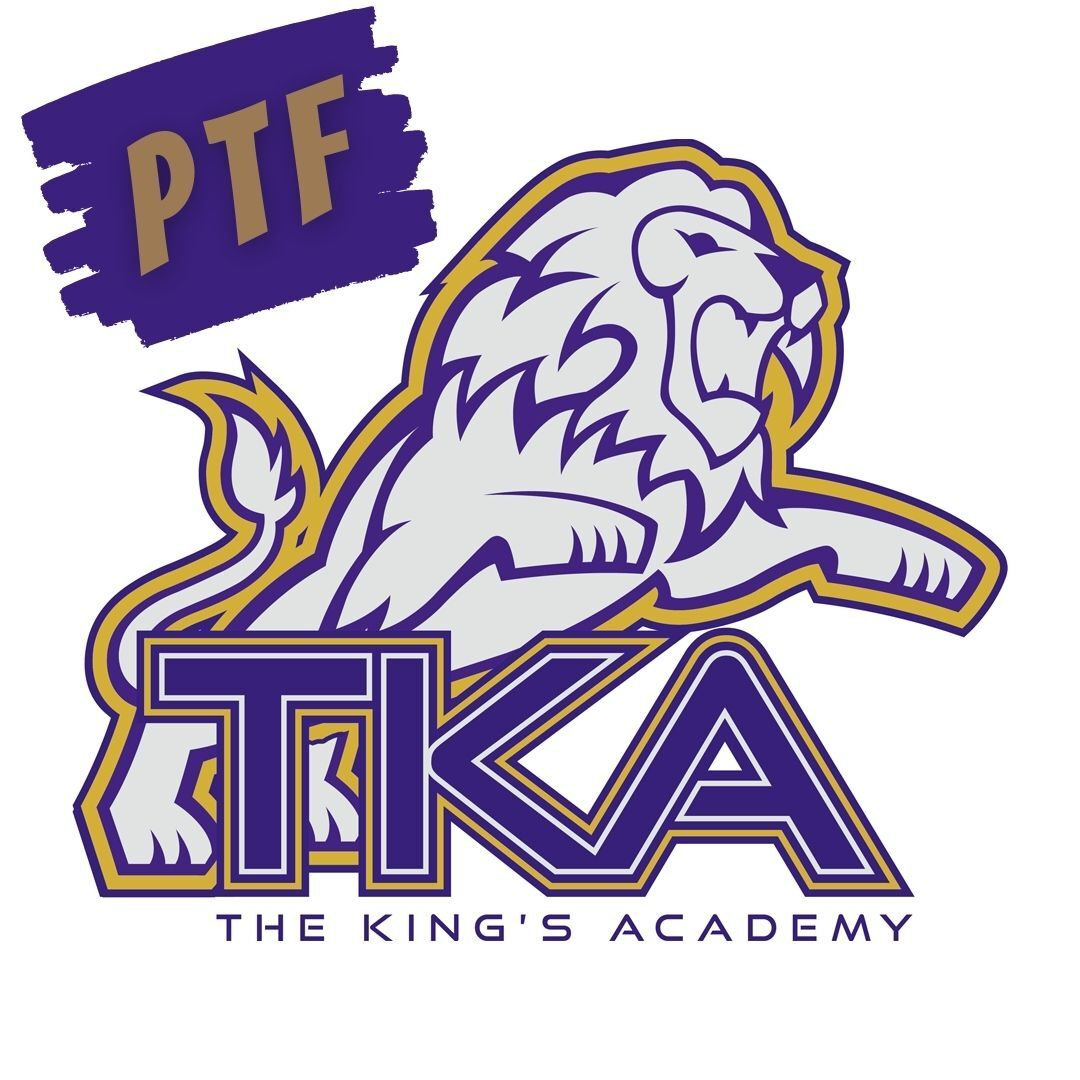# PTF

The next PTF meeting will be tomorrow, February 17, from 3:15 PM-4:05 PM in Brandes.

Childcare will be provided through the aftercare program free of charge until 4:15 PM for the meeting.

If you haven't joined PTF, no worries, come anyway! Membership forms will be available at the meeting. The fee to join is \$25 per family.

We look forward to having you as part of our PTF this year!

 table div table+table+table+table+table+table+table+table+table+table+table+table+table+table+table+table+table+table+table+table+table+table div table{width:100%;padding:0}table div table+table+table+table+table+table+table+table+table+table+table+table+table+table+table+table+table+table+table+table+table+table div table img{width:96.23%;padding:0;float:none}table div table+table+table+table+table+table+table+table+table+table+table+table+table+table+table+table+table+table+table+table+table+table div table td{width:100%;padding:0 1.88% 18px}/* styles */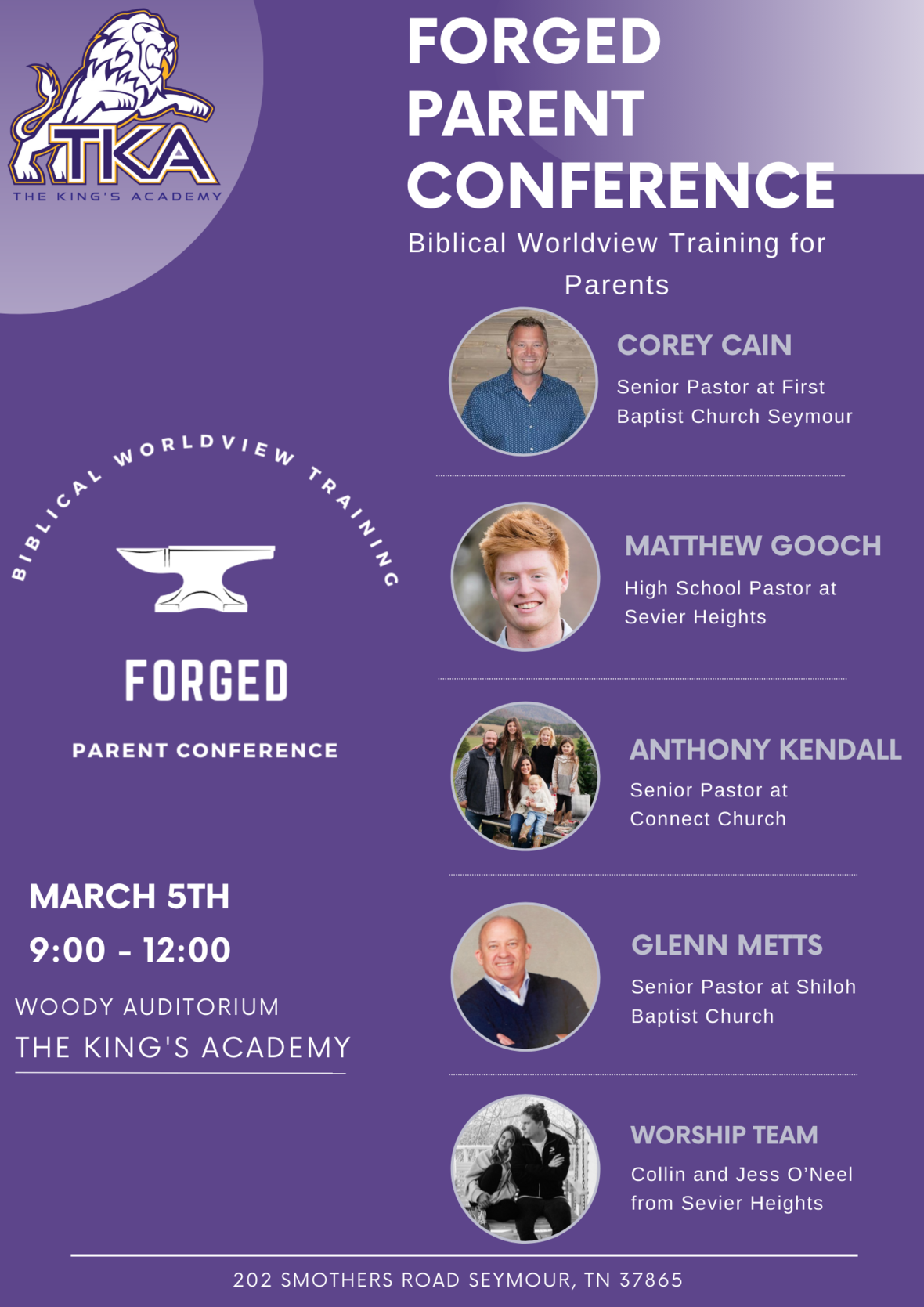# Save The Date

TKA will be hosting the “Forged" Parent Conference on March 5th in Woody Auditorium.

“Forged” will be a morning of biblically-based parenting seminars focused on encouraging parents that are raising students in a post-Christian society. We are blessed to have local ministers from our community coming to share on topics involving discipleship, maneuvering technology in today’s digital society, and student identity in an ever-changing environment. This event is free and will be a great opportunity to link arms with other parents as we focus on what the Bible says about the roles and responsibilities associated with raising children. Join us from 9:00 – 12:00 on Saturday, March 5th for “Forged!”

 table div table+table+table+table+table+table+table+table+table+table+table+table+table+table+table+table+table+table+table+table+table+table+table+table div table{width:100%;padding:0}table div table+table+table+table+table+table+table+table+table+table+table+table+table+table+table+table+table+table+table+table+table+table+table+table div table img{width:96.23%;padding:0;float:none}table div table+table+table+table+table+table+table+table+table+table+table+table+table+table+table+table+table+table+table+table+table+table+table+table div table td{width:100%;padding:0 1.88% 18px}/* styles */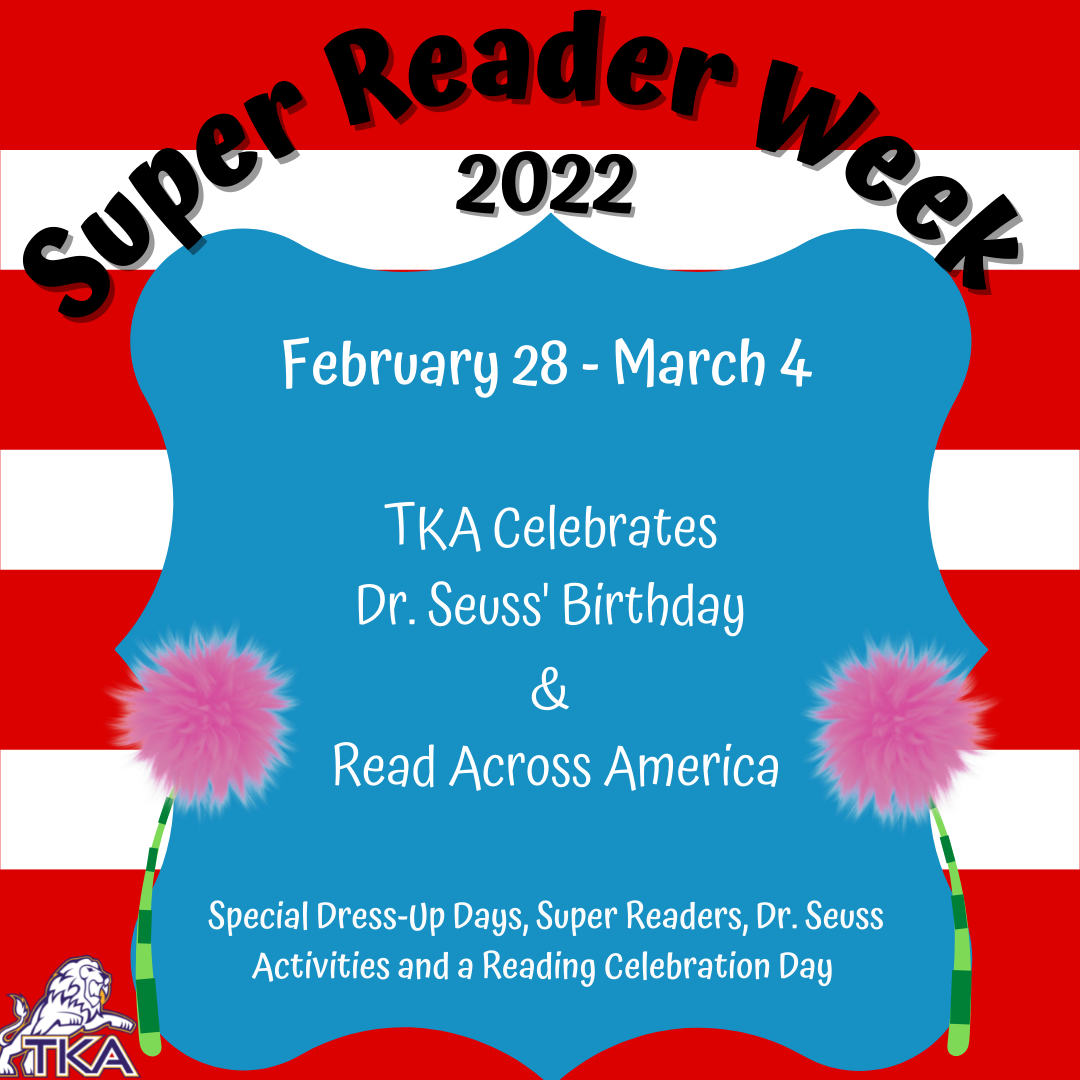We are excited to share with you about our 1st Annual Super Reader Week! Super Reader Week will take place February 28-March 4th. This week coincides with Read Across America Week highlighting Dr. Seuss’ birthday. We have planned a fun filled week highlighting the great importance of reading.

Super Reader Week will include a Dr. Seuss' environment throughout the elementary with decorated doors and hallways, Dr. Seuss Dress Up Days, a special reading time with a Super Reader during library class, special Dr. Seuss activities, and a Reading Celebration Day.

Dr. Seuss Dress Up Days include:
Monday: Fox in Socks- Crazy Sock Day
Tuesday: Green Eggs and Ham- Wear Green
Wednesday: Dr. Seuss’ Birthday- Favorite Dr. Seuss Character
Thursday: Cat in the Hat- Favorite Hat Day
* Friday: Oh, the Places You’ll Go- Favorite college team/jersey

It is a joy to be a part of a school that takes great pride in developing strong readers. We are beyond blessed to share our love for reading with your child(ren) every day. We are looking forward to a super fun week celebrating our little lion readers.

 table div table+table+table+table+table+table+table+table+table+table+table+table+table+table+table+table+table+table+table+table+table+table+table+table+table+table div table{width:100%;padding:0}table div table+table+table+table+table+table+table+table+table+table+table+table+table+table+table+table+table+table+table+table+table+table+table+table+table+table div table img{width:96.23%;padding:0;float:none}table div table+table+table+table+table+table+table+table+table+table+table+table+table+table+table+table+table+table+table+table+table+table+table+table+table+table div table td{width:100%;padding:0 1.88% 18px}/* styles */# Spring Book Fair

The spring book fair will take place during Super Reader Week, February 28-March 4. Students and parents are invited to come and shop at the TKA Library.

Volunteers are also needed during this week to help! If you would be willing to help us, click this link to sign up.

 table div table+table+table+table+table+table+table+table+table+table+table+table+table+table+table+table+table+table+table+table+table+table+table+table+table+table+table+table div table{width:100%;padding:0}table div table+table+table+table+table+table+table+table+table+table+table+table+table+table+table+table+table+table+table+table+table+table+table+table+table+table+table+table div table img{width:96.23%;padding:0;float:none}table div table+table+table+table+table+table+table+table+table+table+table+table+table+table+table+table+table+table+table+table+table+table+table+table+table+table+table+table div table td{width:100%;padding:0 1.88% 18px}/* styles */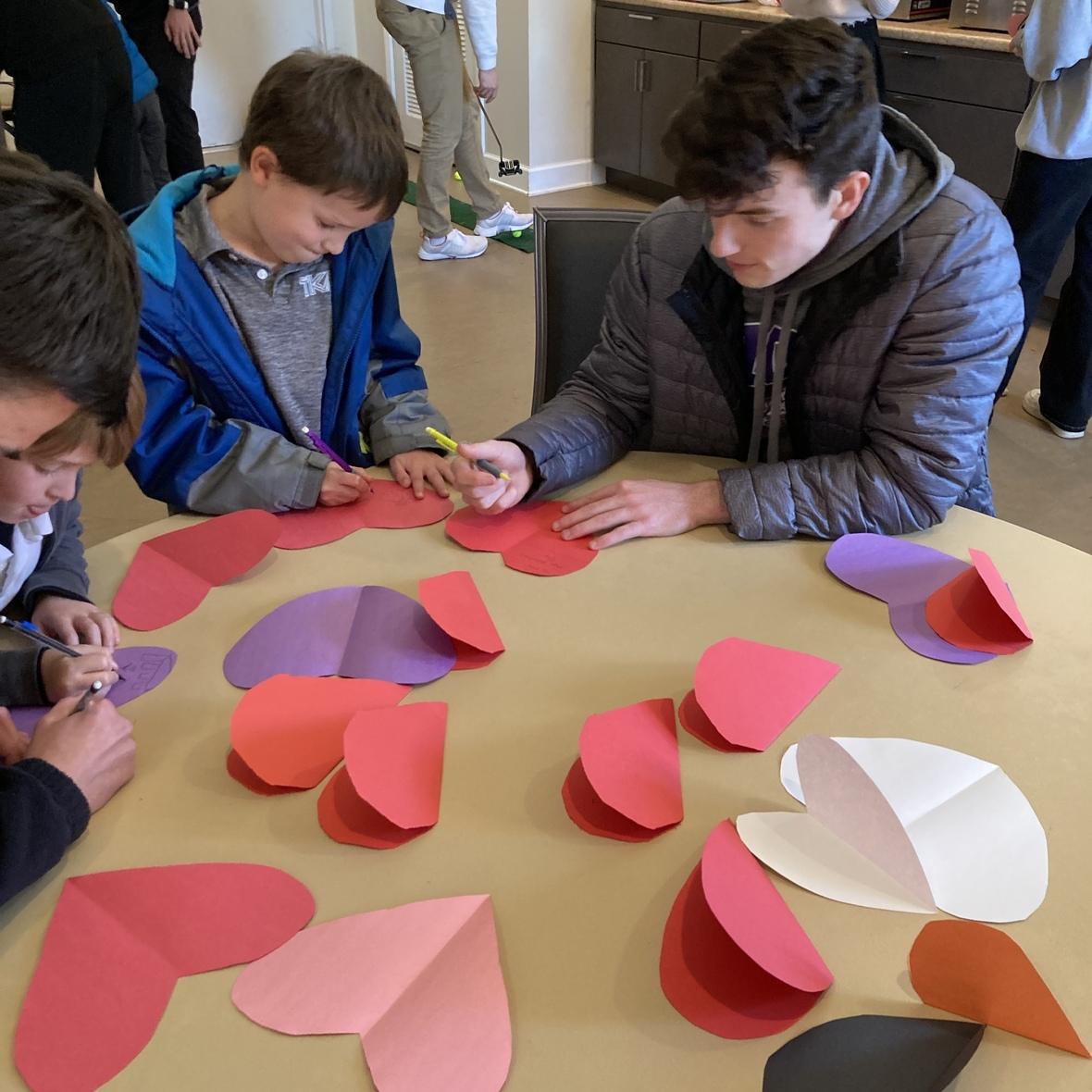# Big and Little Buddy Program

Our Big and Little Buddies celebrated Valentine's Day together with games, crafts, and candy! We love to see the relationship our Seniors and K5 students have formed through this program.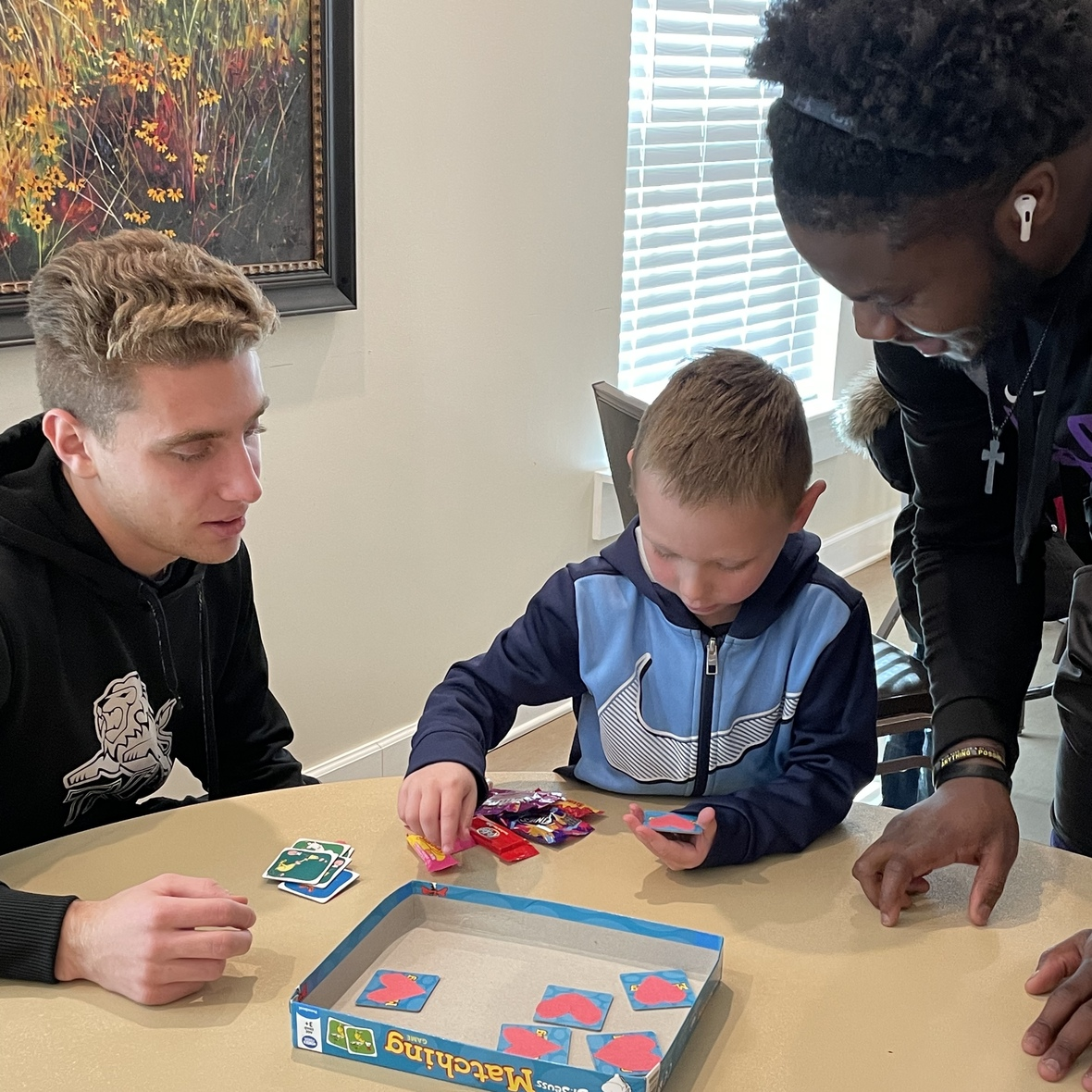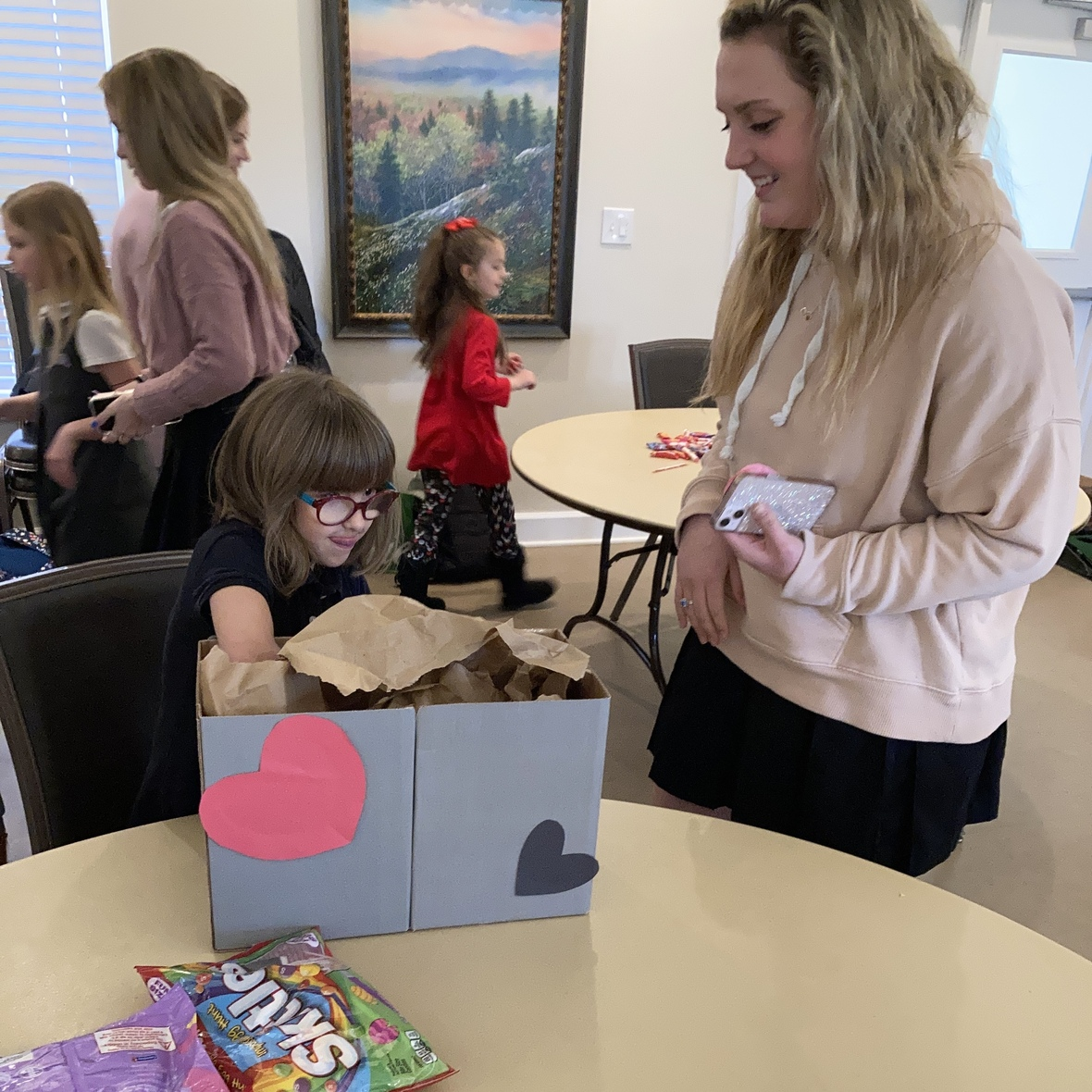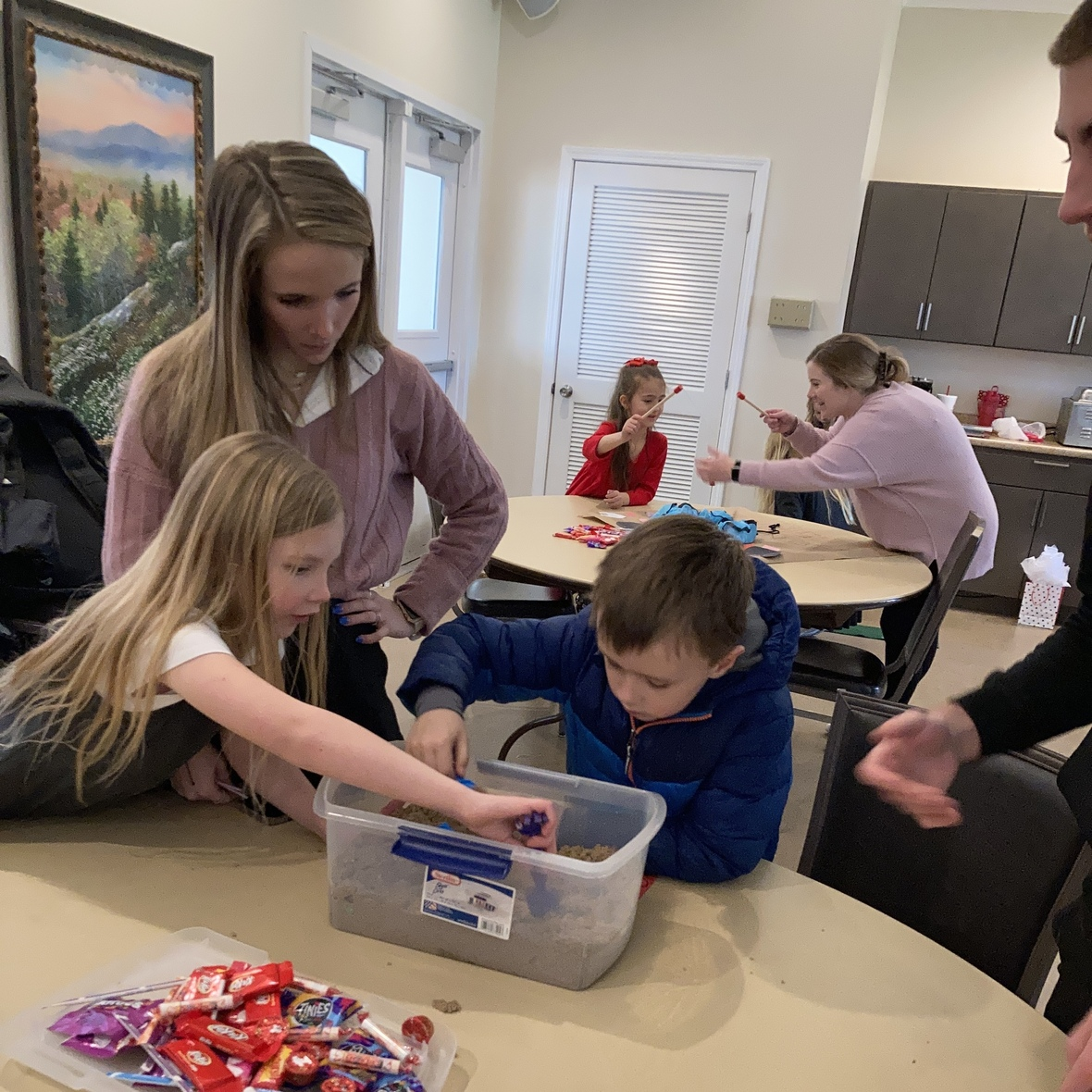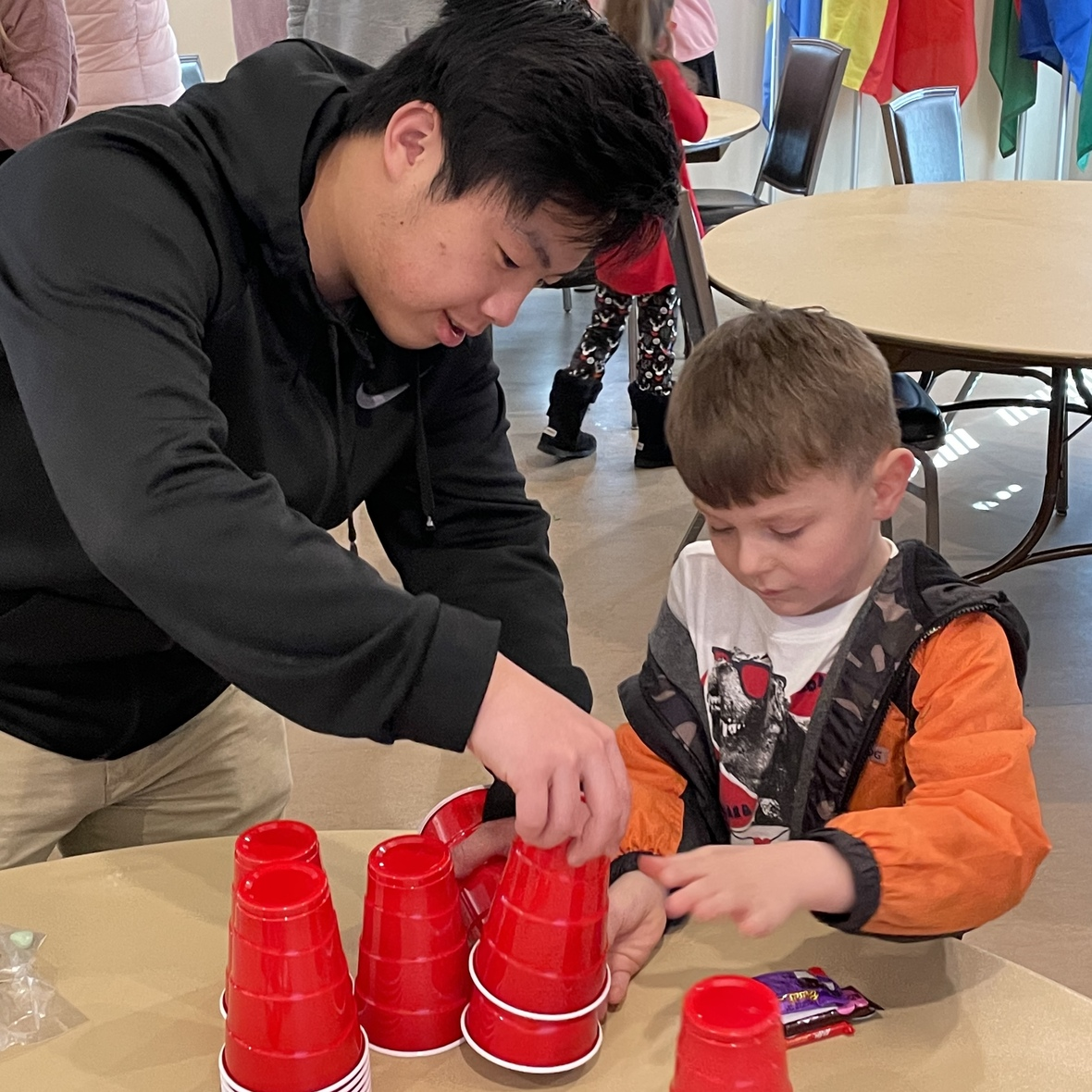table div table+table+table+table+table+table+table+table+table+table+table+table+table+table+table+table+table+table+table+table+table+table+table+table+table+table+table+table+table+table+table+table div table{width:100%;padding:0}table div table+table+table+table+table+table+table+table+table+table+table+table+table+table+table+table+table+table+table+table+table+table+table+table+table+table+table+table+table+table+table+table div table img{width:96.23%;padding:0;float:none}table div table+table+table+table+table+table+table+table+table+table+table+table+table+table+table+table+table+table+table+table+table+table+table+table+table+table+table+table+table+table+table+table div table td{width:100%;padding:0 1.88% 18px}/* styles */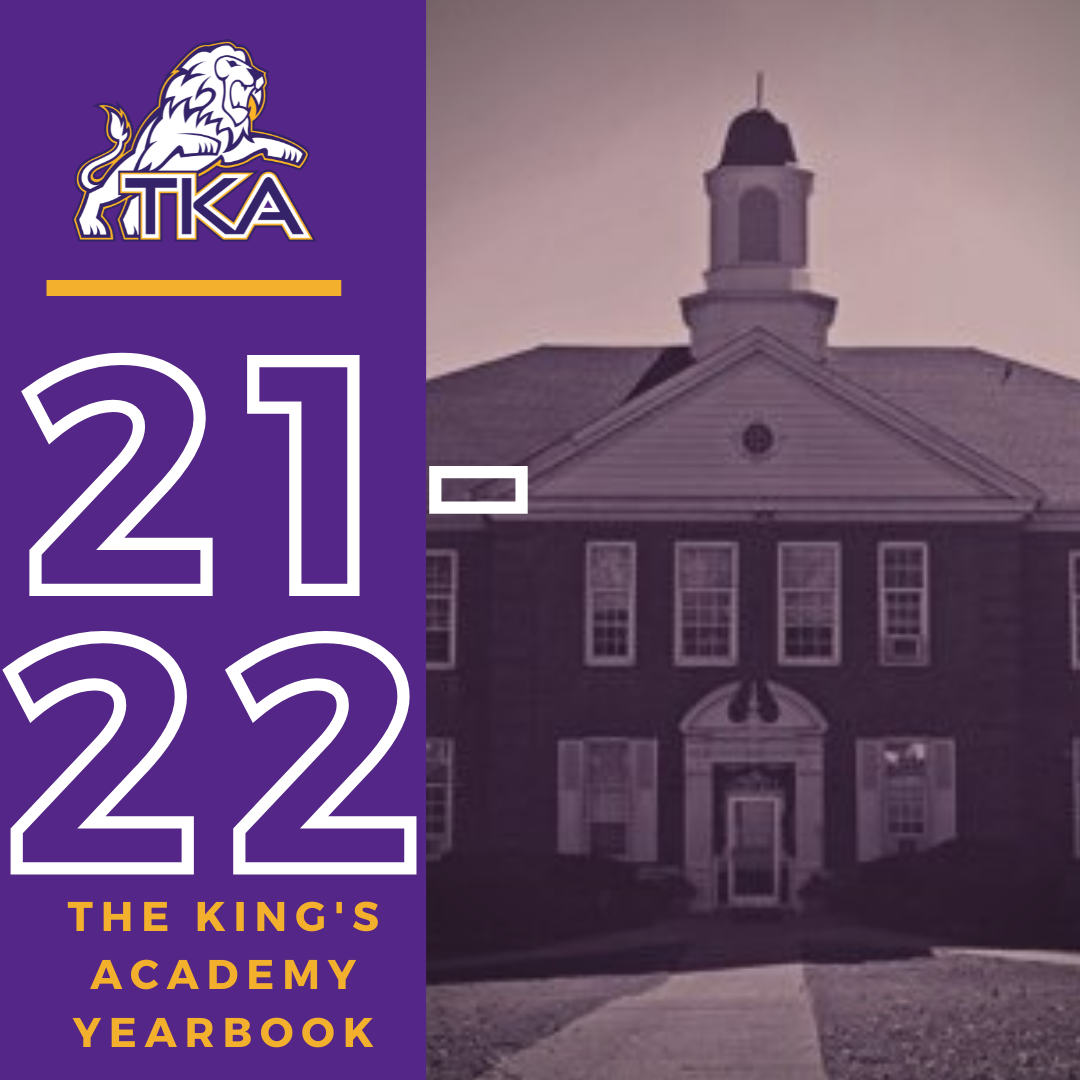# 2021-2022 Yearbook

The price for the 2021-2022 TKA yearbook is \$55. All grades, K3-12 will have the same yearbook. The slight price increase is because we are only going to have one larger all-school book.

All yearbook orders can be placed online--here is the link.Do you have a class of 2022 senior in your household?

Don't miss out on the opportunity to purchase a Senior Tribute for the 2021-2022 yearbook!

Senior Tributes are available for purchase at the bottom of the link listed here.For any questions regarding yearbooks, email Mr. Josh Smith at jsmith@thekingsacademy.net.

 table div table+table+table+table+table+table+table+table+table+table+table+table+table+table+table+table+table+table+table+table+table+table+table+table+table+table+table+table+table+table+table+table+table+table+table+table div table{width:100%;padding:0}table div table+table+table+table+table+table+table+table+table+table+table+table+table+table+table+table+table+table+table+table+table+table+table+table+table+table+table+table+table+table+table+table+table+table+table+table div table img{width:96.23%;padding:0;float:none}table div table+table+table+table+table+table+table+table+table+table+table+table+table+table+table+table+table+table+table+table+table+table+table+table+table+table+table+table+table+table+table+table+table+table+table+table div table td{width:100%;padding:0 1.88% 18px}/* styles *//* styles */
 table div table+table+table+table+table+table+table+table+table+table+table+table+table+table+table+table+table+table+table+table+table+table+table+table+table+table+table+table+table+table+table+table+table+table+table+table+table+table+table div table{width:100%;padding:0}table div table+table+table+table+table+table+table+table+table+table+table+table+table+table+table+table+table+table+table+table+table+table+table+table+table+table+table+table+table+table+table+table+table+table+table+table+table+table+table div table img{width:96.23%;padding:0;float:none}table div table+table+table+table+table+table+table+table+table+table+table+table+table+table+table+table+table+table+table+table+table+table+table+table+table+table+table+table+table+table+table+table+table+table+table+table+table+table+table div table td{width:100%;padding:0 1.88% 18px}/* styles */# Covid-19 Procedures

While we are excited to offer in-person education, we must maintain a safe learning environment for all students, faculty/staff, and additional stakeholders.

To do so, we will be working closely with the Sevier County Health Department regarding Covid-19 protocols.

 table div table+table+table+table+table+table+table+table+table+table+table+table+table+table+table+table+table+table+table+table+table+table+table+table+table+table+table+table+table+table+table+table+table+table+table+table+table+table+table+table+table div table{width:100%;padding:0}table div table+table+table+table+table+table+table+table+table+table+table+table+table+table+table+table+table+table+table+table+table+table+table+table+table+table+table+table+table+table+table+table+table+table+table+table+table+table+table+table+table div table img{width:96.23%;padding:0;float:none}table div table+table+table+table+table+table+table+table+table+table+table+table+table+table+table+table+table+table+table+table+table+table+table+table+table+table+table+table+table+table+table+table+table+table+table+table+table+table+table+table+table div table td{width:100%;padding:0 1.88% 18px}/* styles */table div table+table+table+table+table+table+table+table+table+table+table+table+table+table+table+table+table+table+table+table+table+table+table+table+table+table+table+table+table+table+table+table+table+table+table+table+table+table+table+table+table+table+table div table{width:100%;padding:0}table div table+table+table+table+table+table+table+table+table+table+table+table+table+table+table+table+table+table+table+table+table+table+table+table+table+table+table+table+table+table+table+table+table+table+table+table+table+table+table+table+table+table+table div table img{width:96.23%;padding:0;float:none}table div table+table+table+table+table+table+table+table+table+table+table+table+table+table+table+table+table+table+table+table+table+table+table+table+table+table+table+table+table+table+table+table+table+table+table+table+table+table+table+table+table+table+table div table td{width:100%;padding:0 1.88% 18px}/* styles */